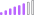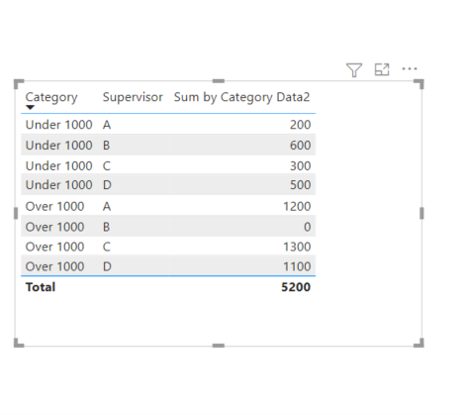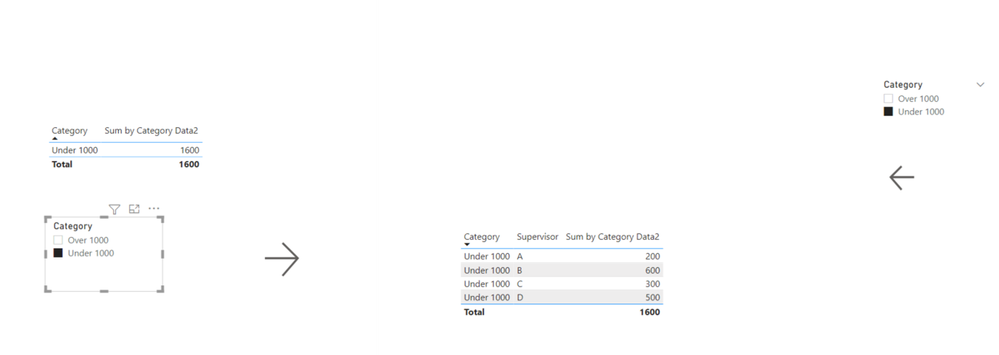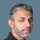cancel
Showing results for
Did you mean:

Fabric is Generally Available. Browse Fabric Presentations. Work towards your Fabric certification with the Cloud Skills Challenge.Post Patron

## Sum of amount by id and categorize after summing

I have data as shown below and I want to sum the amounts by id as shown in Expected sum column

 Id Amount Expected sum 1 100 3300 1 1200 1 100 1 400 1 200 1 1300 2 300 1300 2 1000 3 300 500 3 200

and I want to sum the amounts by Id and show it in categories as below and this is my expected output. It should show the categorization after summing the amounts by id.

 Category Expected_measure Under 1000 500 Over 1000 4600

But I am getting like this when I used a simple SUM measure. It is summing all under 1000 and over 1000 separately and giving this output which is not my expected output.

 Category Sum_measure Under 1000 1600 Over 1000 3500

It should show the categorization after summing the amounts by id.

How do I achieve my expected output?

1 ACCEPTED SOLUTIONSuper User

Hi, @bml123

Please check the below picture and the sample pbix file's link down below.

I have created a separate table, that is the category table looks like below.Sum by Category =
SUMX (
FILTER (
VALUES ( Data[Id] ),
COUNTROWS (
FILTER (
Categories,
CALCULATE ( SUM ( Data[Amount] ) ) >= Categories[Min]
&& CALCULATE ( SUM ( Data[Amount] ) ) < Categories[Max]
)
) > 0
),
CALCULATE ( SUM ( Data[Amount] ) )
)

Hi, My name is Jihwan Kim.

If this post helps, then please consider accept it as the solution to help other members find it faster, and give a big thumbs up.

If this post helps, then please consider accepting it as the solution to help other members find it faster, and give a big thumbs up.

12 REPLIES 12Post Patron

@Jihwan_Kim Thank you so much. It worked perfectly.Community Support

Hi @bml123

You also can try this.

create the measures:

``sum_by_ID = CALCULATE(SUM('Table'[Amount]),FILTER(ALL('Table'),'Table'[Id]=SELECTEDVALUE('Table'[Id])))``
``````M_category =
var _over1000= CALCULATE(SUM('Table'[Amount]),FILTER(ALL('Table'),[sum_by_ID]>1000))
var _under1000=CALCULATE(SUM('Table'[Amount]),FILTER(ALL('Table'),[sum_by_ID]<=1000))
return
if(SELECTEDVALUE(Category[Category])="Over 1000",_over1000,_under1000)``````

Result:Best Regards,

Community Support Team _ Tang

If this post helps, please consider Accept it as the solution to help the other members find it more quickly.Super User

Hi, @bml123

Please check the below picture and the sample pbix file's link down below.

I have created a separate table, that is the category table looks like below.Sum by Category =
SUMX (
FILTER (
VALUES ( Data[Id] ),
COUNTROWS (
FILTER (
Categories,
CALCULATE ( SUM ( Data[Amount] ) ) >= Categories[Min]
&& CALCULATE ( SUM ( Data[Amount] ) ) < Categories[Max]
)
) > 0
),
CALCULATE ( SUM ( Data[Amount] ) )
)

Hi, My name is Jihwan Kim.

If this post helps, then please consider accept it as the solution to help other members find it faster, and give a big thumbs up.

If this post helps, then please consider accepting it as the solution to help other members find it faster, and give a big thumbs up.Post Patron

I have to show the same breakdown by Supervisor level.  Can you please let me know how do I acheieve that?Super User

Hi, @bml123

In the sample file, I only can see Id, amount, and category.

Can you please let me know how to break it down by supervisor level?

If this post helps, then please consider accepting it as the solution to help other members find it faster, and give a big thumbs up.Post Patron

Here is my data

 Supervisor Id Amount A 1 100 A 1 1200 A 1 100 B 1 400 B 1 200 C 1 1300 C 2 300 D 2 1100 D 3 300 D 3 200

and I want to show the breakdown like this.  How do I achieve this?

 Under 1000 A 200 Under 1000 B 600 Under 1000 C 300 Under 1000 D 500 Over 1000 A 1200 Over 1000 B Over 1000 C 1300 Over 1000 D 1100Super User

Hi, @bml123

I am not sure if I understood your table correctly, but because the logic is a little different than the first question's logic, so I needed to add an index column to differentiate the first row and the third row.If this post helps, then please consider accepting it as the solution to help other members find it faster, and give a big thumbs up.Post Patron

@Jihwan_Kim Thank you for the response. I would like to show the details when right clicked and drill through is selected.  As the categories table is not connected to any other table in the data model, how can we show the correct details when drill through is clickedSuper User

Hi, @bml123

I am not sure if I understood your question correctly.If this post helps, then please consider accepting it as the solution to help other members find it faster, and give a big thumbs up.Post Patron

In your first table, if I add supervisor, and if I right click on supervisor, it should take me to the details page and show the details of that supervisor only. Is that possible to do? the details page and category table are not related. Not sure if that is possible.

is there a way to create the measures in the details table itself without creating them in a separate category table so that it is easier to drill through to the details page.Post Patron

is there a way to create the measures in the details table itself without creating them in a separate category table so that it is easier to drill through to the details page.Super User

@bml123

Create two measures for Under and Above 1000 and insert them in a matrix and turn on "Show on Rows" in the properties.

``````Above 1000 =

var __IdAmount =
SUMMARIZE( Table6, Table6[Id] ),
"Total", CALCULATE(SUM(Table6[Amount]))
)
return
SUMX(__IdAmount, ([Total] >= 1000 ) * [Total]) ``````
``````Under 1000 =

var __IdAmount =
SUMMARIZE( Table6, Table6[Id] ),
"Total", CALCULATE(SUM(Table6[Amount]))
)
return
SUMX(__IdAmount, ([Total] < 1000 ) * [Total]) ``````Did I answer your question? Mark my post as a solution! and hit thumbs upAnnouncements#### Power BI Monthly Update - November 2023

Check out the November 2023 Power BI update to learn about new features.#### Fabric Community News unified experience

Read the latest Fabric Community announcements, including updates on Power BI, Synapse, Data Factory and Data Activator.#### Exclusive opportunity for Women!

Join us for a free, hands-on Microsoft workshop led by women trainers for women where you will learn how to build a Dashboard in a Day!#### The largest Power BI and Fabric virtual conference

130+ sessions, 130+ speakers, Product managers, MVPs, and experts. All about Power BI and Fabric. Attend online or watch the recordings.Top Solution Authors
Top Kudoed Authors
Users online (5,823)